• svm预测模型matlab
千次阅读
2021-04-24 19:25:28

我把14章，SVM用于回归建模的例子改动后，结果出现了问题：

首先，我描述一下，我想做的东西：

我要预测输出的压力，当前的输出的压力和前3个时刻的5个变量有关，我也想减少变量，可是预测的效果就不好了)。我将程序改动如下，同时我出现几个问题：

【1】我用第 208  209 210 个时刻的数据 作为输入数据，来预测211时刻的输出压力。但由于我编写的程序，输出总能得到3个，也就是可以预测 211 212 213 的输出压力。

刚开始，我是这么想的，212时刻的输出按我的本意的话，应该是知道了 209 210 211时刻的输入后才能预测到的，这个时候预测的肯定是错误的，不用理会。但后来我发现，    当我把输入改成209 210  211 时刻是，我发现我错了，因为此时给出的212  213 214 这3个输出数据中，212 213 和上面将 208 209 210作为输入时预测到的数据一摸一样。所以我的模型应该是错误的，可我看不出来。我想让作者给我看看。我把程序贴到下面了。

%% SVM神经网络的回归预测分析---压缩机四段出口压力预测

%% 清空环境变量

function output_press

tic;

close all;

clear;

clc;

format compact;

%% 数据的提取和预处理

train_x = data(1:210,1:5);

train_y = data(4:213,3);

test_x = data(211:216,1:5);

test_y = data(214:219,3)

% 数据预处理,将原始数据进行归一化

%

[train_x_scale,test_x_scale] = scaleForSVM(train_x,test_x,-1,1);

[train_y_scale,test_y_scale,ps] = scaleForSVM(train_y,test_y,-1,1);

%% 参数c和g寻优选择

% %选择回归预测分析最佳的SVM参数c&g

%%粒子群算法优化

pso_option.c1 = 2.0;

pso_option.c2 =0.6;

pso_option.maxgen = 200;

pso_option.sizepop = 20;

pso_option.k = 1.2;

pso_option.wV = 1;

pso_option.wP = 1;

pso_option.v = 5;

pso_option.popcmax = 100;

pso_option.popcmin = 0.0001;

pso_option.popgmax = 1000;

pso_option.popgmin = 0.0001;

[bestmse,bestc,bestg] = psoSVMcgForRegress(train_y_scale,train_x_scale,pso_option);

%打印精细选择结果

disp('打印精细选择----粒子群算法优化结果');

str = sprintf( 'Best Cross Validation MSE = %g Best c = %g Best g = %g',bestmse,bestc,bestg);

disp(str);

%% 利用回归预测分析最佳的参数进行SVM网络训练

cmd = ['-c ', num2str(bestc), ' -g ', num2str(bestg) , ' -s 3 -t 2 -p 0.01'];

model = svmtrain(train_y_scale,train_x_scale,cmd);

%% SVM网络回归预测

%用原来的训练数据来看系统的预测能力如何

[predict_train,mse] = svmpredict(train_y_scale,train_x_scale,model);

predict_train= mapminmax('reverse',predict_train',ps);

predict_train=predict_train';

% %打印回归结果

str = sprintf( '原训练数据——均方误差 MSE = %g 相关系数 R = %g%%',mse(2),mse(3)*100);

disp(str);

figure;

hold on;

plot(train_y,'-o');

plot(predict_train,'g-*');

legend('原始数据','回归预测数据');

hold off;

title('原始数据和回归预测数据对比','FontSize',12);

xlabel('压缩机运行时间(2011.2.15.15时-2011.02.25.11时)','FontSize',12);

ylabel('压缩机四段出口压力','FontSize',12);

grid on;

figure;

error = predict_train - test_y;

plot(error,'rd');

title('误差图(predicted data - original data)','FontSize',12);

xlabel('压缩机运行时间(2011.2.15.15时-2011.02.25.11时)','FontSize',12);

ylabel('误差量','FontSize',12);

grid on;

figure;

error = (predict_train- test_y)./test_y;

plot(error,'rd');

title('相对误差图(predicted data - original data)/original data','FontSize',12);

xlabel('压缩机运行时间(2011.2.15.15时-2011.02.25.11时)','FontSize',12);

ylabel('相对误差量','FontSize',12);

grid on;

snapnow;

toc;

save output_press_3.mat model

【2】如果您比较忙的话，您大致给我说说思路，就是用前3个时刻来预测当前这个时刻，怎么预测呢?/

[本帖最后由 史峰 于 2011-7-29 12:45 编辑]

更多相关内容
• MATLAB源码集锦-基于SVM支持向量机算法的降水量预测模型代码
• 预测模型-SVM预测】基于SVM实现股票趋势预测matlab源码.zip简介
• 1 模型 基于数据的机器学习就是由观测样本数据得出目前尚不能通过原理分析得到的规律,利用其对未来数据进行预测.神经网络以其优越的函数逼近性能广泛用于建立时间序列过去与未来数据之间某种确定的映射关系,实现...

## 1 模型

基于数据的机器学习就是由观测样本数据得出目前尚不能通过原理分析得到的规律,利用其对未来数据进行预测.神经网络以其优越的函数逼近性能广泛用于建立时间序列过去与未来数据之间某种确定的映射关系,实现预测.首先分析了以经验风险最小化为准则的神经网络的局限性,以及针对此提出的结构风险最小化准则的优点;其次引出支持向量机;最后利用支持向量机对股票数据做较准确的多步预测.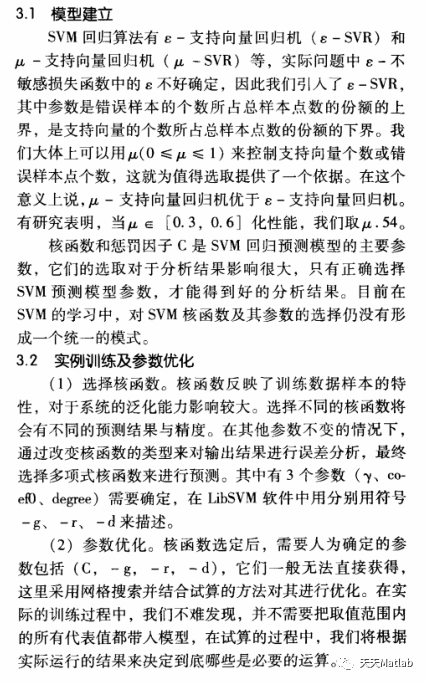## 2 部分代码

clear all;

clc ;

close all

%得到文件路径，找到所有.dat格式的文件

%extract data

[m,n] = size(sh);

n1 = round(length(sh)*0.9);%训练样本大小

T=n1+1:m;%预测天数

ts = sh(1:n1,1);%训练数据

tsx = sh(n1+1:m,1);%测试数据

original = sh(n1+1:end,:);%原始测试数据

%归一化处理

[TS,TSps] = mapminmax(ts);

[TSX,TSXps] = mapminmax(tsx);

[TSX_zong,TSXps_zong] = mapminmax(sh);

%split the data into training and testing

train_label = TS(1:n1,:);%训练数据标签

train_data = TS(1:n1,:);%训练数据

test_label = TSX(1:end,:);%测试数据标签

test_data = TSX(1:end,:);%测试数据

test_label_zong = TSX_zong(1:end,:);%测试数据标签

test_data_zong = TSX_zong(1:end,:);%测试数据

% Find the optimize value of c,g paramter

% Approximately choose the parameters:

% the scale of c is 2^(-5),2^(-4),...,2^(10)

% the scale of g is 2^(-5),2^(-4),...,2^(5)

[bestmse,bestc,bestg] = svmregress(train_label,train_label,-5,10,-5,5,3,1,1,0.0005);

% Display the approximate result

disp('Display the approximate result');

str = sprintf( 'Best Cross Validation MSE = %g Best c = %g Best g = %g',bestmse,bestc,bestg);

disp(str);

%Do training by using svmtrain of libsvm

cmd = ['-c ', num2str(bestc), ' -g ', num2str(bestg) , ' -s 3 -p 0.01'];

model = svmtrain(train_label,train_data,cmd);%训练

%Do predicting by using svmpredict of libsvm

predict= svmpredict(test_label,test_data,model);%预测

predict = mapminmax('reverse',predict,TSXps);%反归一化

predict_zong= svmpredict(test_label_zong,test_data_zong,model);%预测

predict_zong = mapminmax('reverse',predict_zong,TSXps_zong);%反归一化

% Display the result of SVM Regression

% str = sprintf( '误差均方差MSE = %g R = %g%%',mse(2),mse(3)*100);

disp(str);

figure(1);

plot(sh,'k*-','LineWidth',2);

hold on;

plot(predict_zong,'ro-','LineWidth',2);

legend('原始数据','预测数据');

xlabel('时刻')

ylabel('用电负荷')

title('总体预测')

hold off;

figure(2);

plot(T,original,'b*-','LineWidth',1);

hold on;

plot(T,predict,'ro-','LineWidth',1);

xlabel('时刻')

ylabel('用电负荷')

legend('原始数据','预测数据');

title('未来几天预测')

hold off;

figure(3);

plot(T,original-predict,'bo-','LineWidth',1);

title('未来几天预测误差图')

xlabel('时刻')

ylabel('用电负荷')

hold off;

## 3 仿真结果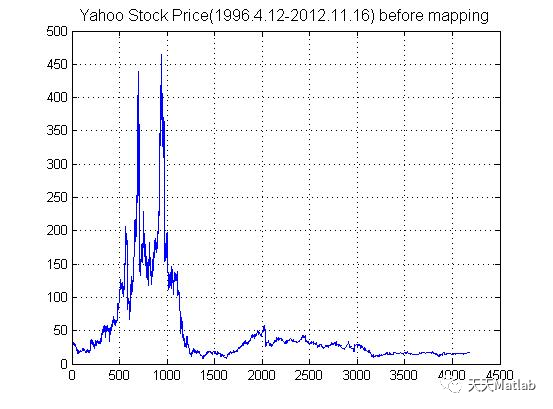## 4 参考文献

杨一文, and 杨朝军. "基于支持向量机的金融时间序列预测." 系统管理学报 14.2(2005):176-181.

## 5 MATLAB代码与数据下载地址

见博客主页

展开全文matlab 机器学习 神经网络
• svm回归预测matlab代码用于链接预测的Weisfeiler-Lehman神经机器 用法 Wesfeiler-Lehman神经机器（WLNM）是一种基于子图的链接预测方法，该方法利用深度学习自动学习图结构特征，以便根据链接的封闭子图进行链接预测...支持向量机
• % SVM模型训练 %% SVM网络回归预测 [output_test_pre,acc,decision_values]=svmpredict(output_test',input_test',model_cs_svr); % SVM模型预测及其精度 test_pre=mapminmax('reverse',output_test_pre',rule2); ...

## 一、布谷鸟算法简介

布谷鸟算法，英文叫做Cuckoo search (CS algorithm)。首先还是同样，介绍一下这个算法的英文含义， Cuckoo是布谷鸟的意思，啥是布谷鸟呢，是一种叫做布谷的鸟，o(∩_∩)o ，这种鸟她妈很懒，自己生蛋自己不养，一般把它的宝宝扔到别的种类鸟的鸟巢去。但是呢，当孵化后，遇到聪明的鸟妈妈，一看就知道不是亲生的，直接就被鸟妈妈给杀了。于是这群布谷鸟宝宝为了保命，它们就模仿别的种类的鸟叫，让智商或者情商极低的鸟妈妈误认为是自己的亲宝宝，这样它就活下来了。
布谷鸟搜索算法（Cuckoo Search, CS）是2009年Xin-She Yang 与Suash Deb在《Cuckoo Search via Levy Flights》一文中提出的一种优化算法。布谷鸟算法是一种集合了布谷鸟巢寄生性和莱维飞行（Levy Flights）模式的群体智能搜索技术，通过随机游走的方式搜索得到一个最优的鸟巢来孵化自己的鸟蛋。这种方式可以达到一种高效的寻优模式。

1 布谷鸟的巢寄生性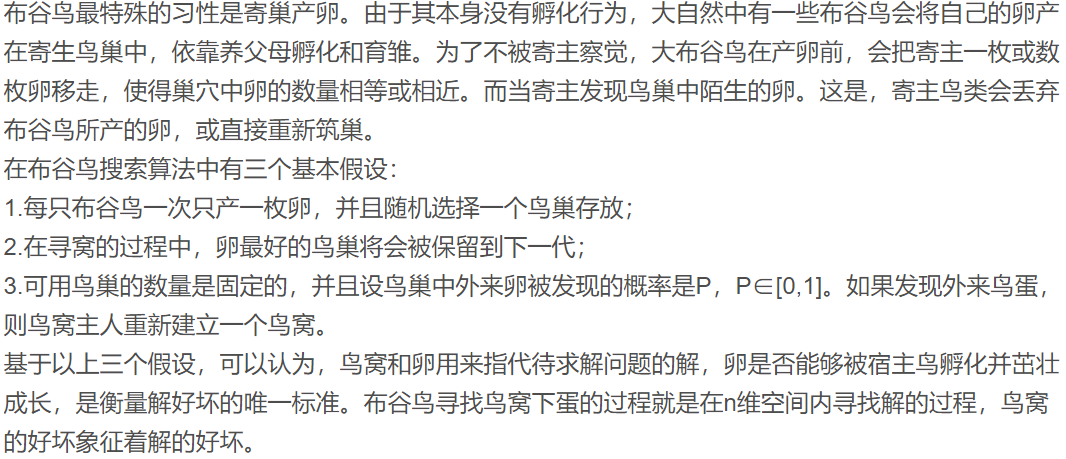2 莱维飞行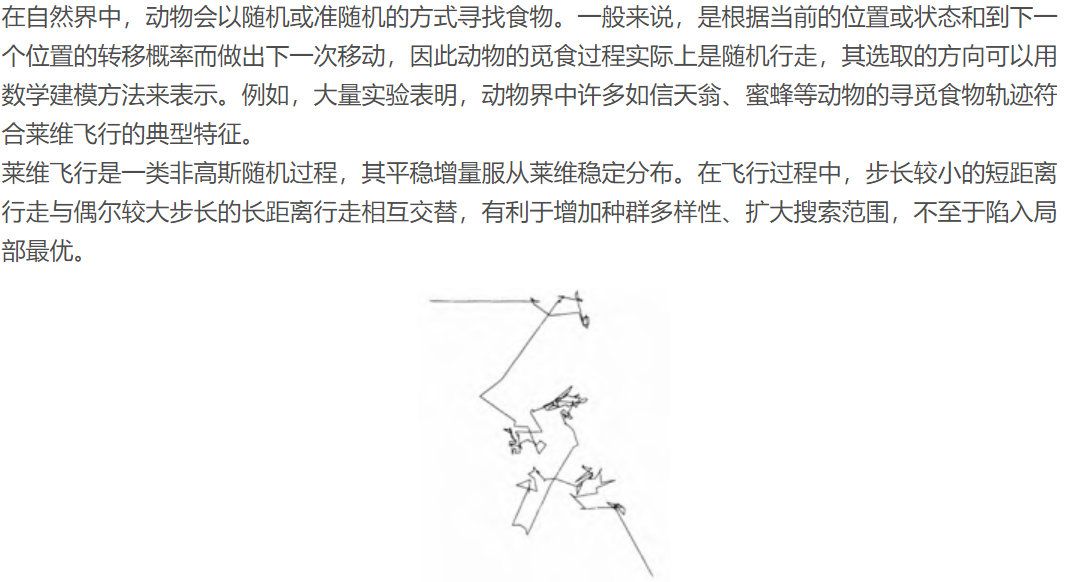图1.模拟莱维飞行轨迹示意图

3 布谷鸟搜索算法的实现过程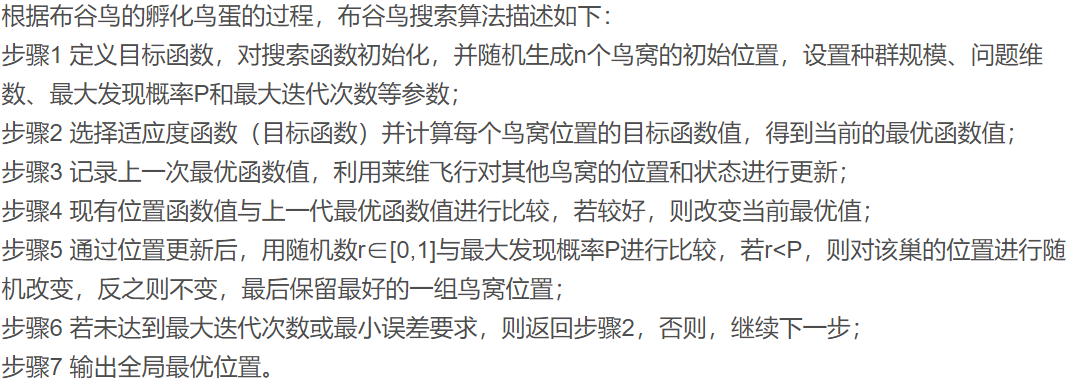## 二、部分源代码

clear; clc; close all;
%% 数据导入
IMF = data(:,14);
%% 划分训练集和测试集
x = 5; % sliding window length
z = 1; % output length
[train_input,train_output,test_input,test_output] = Split(IMF,x,z); % 默认按照 8:2 的比例划分训练集和测试集
%% 预处理
%归一化

%% CS-SVR
time= 50;
n=20; % n为巢穴数量
pa=0.20; % 被宿主发现的概率
dim = 2; % 需要寻优的参数个数

% 随机初始化巢穴
nest=zeros(n,dim);
for i=1:n % 遍历每个巢穴
nest(i,:)=Lb+(Ub-Lb).*rand(size(Lb)); % 对每个巢穴，随机初始化参数
end

fitness=ones(1,n); % 目标函数值初始化
[fmin,bestnest,nest,fitness]=get_best_nest(nest,nest,fitness,input_train,output_train,input_test,output_test); % 找出当前最佳巢穴和参数

%% 迭代开始
for t=1:time
new_nest=get_cuckoos(nest,bestnest,Lb,Ub); % 保留当前最优解，寻找新巢穴
[~,~,nest,fitness]=get_best_nest(nest,new_nest,fitness,input_train,output_train,inpu
% 找出当前最佳巢穴和参数
[fnew,best,nest,fitness]=get_best_nest(nest,new_nest,fitness,input_train,output_train,input_test,output_test);
if fnew<fmin
fmin=fnew;
bestnest=best ;
end
end
%% 打印参数选择结果
bestobjfun=fmin;
bestc=bestnest(1);
bestg=bestnest(2);
disp('打印参数选择结果');
str=sprintf('Best c = %g，Best g = %g',bestc,bestg);
disp(str)

%% 利用回归预测分析最佳的参数进行SVM网络训练
cmd_cs_svr=['-s 3 -t 2',' -c ',num2str(bestnest(1)),' -g ',num2str(bestnest(2))];
model_cs_svr = svmtrain(output_train',input_train',cmd_cs_svr); % SVM模型训练

%% SVM网络回归预测
[output_test_pre,acc,decision_values]=svmpredict(output_test',input_test',model_cs_svr); % SVM模型预测及其精度
test_pre=mapminmax('reverse',output_test_pre',rule2);
test_pre = test_pre';

figure('Name','原始-预测图')
plot(test_pre,'r-');hold on;plot((test_output),'b-');
legend('预测','原始')
set(gcf,'unit','centimeters','position',[15,13,20,13])

result=[test_output',test_pre];

MAE = mymae(test_output',test_pre)
MSE = mymse(test_output',test_pre)
MAPE = mymape(test_output',test_pre)
%% 显示程序运行时间
% toc
function [bestsol,fval]=cuckoo_ori_with_chinese_note(time)
% 由CS算法源码添加中文注释，Genlovy Hoo，2016.09.05

clear
clc
close all
format long

if nargin<1
% Number of iteraions 迭代次数
time=2000;
end

disp('Computing ... it may take a few minutes.');

% Number of nests (or different solutions)
n=25; % n为巢穴数量
% Discovery rate of alien eggs/solutions
pa=0.25; % 被宿主发现的概率

% Simple bounds of the search domain
% Lower bounds and upper bounds
dim = 3; % 需要寻优的参数个数
Lb=[0.05,0.25,2.0]; % 设置参数下界
Ub=[2.0,1.3,15.0]; % 设置参数上界

% Random initial solutions
nest=zeros(n,dim);
for i=1:n % 遍历每个巢穴
nest(i,:)=Lb+(Ub-Lb).*rand(size(Lb)); % 对每个巢穴，随机初始化参数
end

% Get the current best
fitness=10^10*ones(n,1); % 目标函数值初始化
[fmin,bestnest,nest,fitness]=get_best_nest(nest,nest,fitness); % 找出当前最佳巢穴和参数

N_iter=0; % 迭代计数器
%% Starting iterations
for t=1:time

% Generate new solutions (but keep the current best)
new_nest=get_cuckoos(nest,bestnest,Lb,Ub); % 保留当前最优解，寻找新巢穴
[fnew,best,nest,fitness]=get_best_nest(nest,new_nest,fitness); % 找出当前最佳巢穴和参数
% Update the counter
N_iter=N_iter+n; % 更新计数器
% Discovery and randomization
new_nest=empty_nests(nest,Lb,Ub,pa); % 发现并更新劣质巢穴

% Evaluate this solution
[fnew,best,nest,fitness]=get_best_nest(nest,new_nest,fitness); % 找出当前最佳巢穴和参数
% Update the counter again
N_iter=N_iter+n; % 更新计数器

end %% End of iterations

%% Post-optimization processing
%% Display all the nests
disp(strcat('Total number of iterations=',num2str(N_iter)));
fmin
bestnest

%% --------------- All subfunctions are list below ------------------
%% Get cuckoos by ramdom walk 通过随机游走搜寻鸟巢
function nest=get_cuckoos(nest,best,Lb,Ub)
% Levy flights
n=size(nest,1); % 鸟巢个数
% Levy exponent and coefficient
% For details, see equation (2.21), Page 16 (chapter 2) of the book
% X. S. Yang, Nature-Inspired Metaheuristic Algorithms, 2nd Edition, Luniver Press, (2010).

% Levy flights参数准备
beta=3/2;
sigma=(gamma(1+beta)*sin(pi*beta/2)/(gamma((1+beta)/2)*beta*2^((beta-1)/2)))^(1/beta); % gamma(x)求gamma函数值

for j=1:n % 遍历每个巢穴
s=nest(j,:); % 提取当前巢穴的参数
% This is a simple way of implementing Levy flights
% For standard random walks, use step=1;
%% Levy flights by Mantegna's algorithm
u=randn(size(s))*sigma; % 生成服从 N(0,sigma^2) 的随机数u，u为长度为参数个数的向量
v=randn(size(s)); % 生成服从 N(0,1) 的随机数v向量
step=u./abs(v).^(1/beta); % 计算步长

% In the next equation, the difference factor (s-best) means that
% when the solution is the best solution, it remains unchanged.
stepsize=0.01*step.*(s-best); % 巢穴位置变化量，如当前巢穴为最优解，则变化量将为0
% Here the factor 0.01 comes from the fact that L/100 should the typical
% step size of walks/flights where L is the typical lenghtscale;
% otherwise, Levy flights may become too aggresive/efficient,
% which makes new solutions (even) jump out side of the design domain
% (and thus wasting evaluations).
% Now the actual random walks or flights
s=s+stepsize.*randn(size(s)); % 步长调整
% Apply simple bounds/limits
nest(j,:)=simplebounds(s,Lb,Ub); % 更新巢穴
end



## 三、运行结果## 四、matlab版本及参考文献

1 matlab版本
2014a

2 参考文献
 包子阳,余继周,杨杉.智能优化算法及其MATLAB实例（第2版）[M].电子工业出版社，2016.
张岩,吴水根.MATLAB优化算法源代码[M].清华大学出版社，2017.
周品.MATLAB 神经网络设计与应用[M].清华大学出版社，2013.
陈明.MATLAB神经网络原理与实例精解[M].清华大学出版社，2013.
方清城.MATLAB R2016a神经网络设计与应用28个案例分析[M].清华大学出版社，2018.

展开全文matlab 支持向量机 算法
• 资源名：做时间序列预测的svm程序_matlab_多种时间序列预测模型 资源类型：matlab项目全套源码 源码说明： 全部项目源码都是经过测试校正后百分百成功运行的，如果您下载后不能运行可联系我进行指导或者更换。 适合...
• 预测模型】基于SVM电力系统短期负荷预测matlab源码.md
• 生物学家Craig Reynolds在1987年提出了一个非常有影响的鸟群聚集模型，在他的仿真中，每一个个体都遵循：避免与邻域个体相撞：匹配邻域个体的速度；飞向鸟群中心，且整个群体飞向目标。仿真中仅利用上面三条简单的...

## 一、粒子群算法简介

1 引言
自然界中的鸟群和鱼群的群体行为一直是科学家的研究兴趣所在。生物学家Craig Reynolds在1987年提出了一个非常有影响的鸟群聚集模型，在他的仿真中，每一个个体都遵循：避免与邻域个体相撞：匹配邻域个体的速度；飞向鸟群中心，且整个群体飞向目标。仿真中仅利用上面三条简单的规则，就可以非常接近地模拟出鸟群飞行的现象。1990年， 生物学家Frank Heppner也提出了鸟类模型， 它的不同之处在于：鸟类被吸引飞到栖息地。在仿真中，一开始每一只鸟都没有特定的飞行目标，只是使用简单的规则确定自己的飞行方向和飞行速度，当有一只鸟飞到栖息地时，它周围的鸟也会跟着飞向栖息地，最终整个鸟群都会落在栖息地。
1995年， 美国社会心理学家James Kennedy和电气工程师RussellEberhart共同提出了粒子群算法(ParticleS warm Optimization， PSO) ， 该算法的提出是受对鸟类群体行为进行建模与仿真的研究结果的启发。他们的模型和仿真算法主要对Frank Heppner的模型进行了修正，以使粒子飞向解空间并在最优解处降落。粒子群算法一经提出，由于其算法简单，容易实现，立刻引起了进化计算领域学者们的广泛关注， 形成一个研究热点。2001年出版的J.Kennedy与R.Eberhart合著的《群体智能》将群体智能的影响进一步扩大[] ， 随后关于粒子群优化算法的研究报告和研究成果大量涌现，继而掀起了国内外研究热潮[2-7]。
粒子群优化算法来源于鸟类群体活动的规律性，进而利用群体智能建立一个简化的模型。它模拟鸟类的觅食行为，将求解问题的搜索空间比作鸟类的飞行空间，将每只鸟抽象成一个没有质量和体积的粒
子，用它来表征问题的一个可能解，将寻找问题最优解的过程看成鸟类寻找食物的过程，进而求解复杂的优化问题。粒子群优化算法与其他进化算法一样，也是基于“种群”和“进化”的概念，通过个体间
的协作与竞争，实现复杂空间最优解的搜索。同时，它又不像其他进化算法那样对个体进行交叉、变异、选择等进化算子操作，而是将群体中的个体看作在l维搜索空间中没有质量和体积的粒子，每个粒子以一定的速度在解空间运动， 并向自身历史最佳位置P best和邻域历史最佳位置g best聚集， 实现对候选解的进化。粒子群算法具有很好的生物社会背景而易于理解，由于参数少而容易实现，对非线性、多峰问题均具有较强的全局搜索能力，在科学研究与工程实践中得到了广泛关注。目前，该算法已广泛应用于函数优化、神经网络训练、模式分类、模糊控制等领域。

2 粒子群算法理论
2.1粒子群算法描述
鸟类在捕食过程中，鸟群成员可以通过个体之间的信息交流与共享获得其他成员的发现与飞行经历。在食物源零星分布并且不可预测的条件下，这种协作机制所带来的优势是决定性的，远远大于对食物
的竞争所引起的劣势。粒子群算法受鸟类捕食行为的启发并对这种行为进行模仿，将优化问题的搜索空间类比于鸟类的飞行空间，将每只鸟抽象为一个粒子，粒子无质量、无体积，用以表征问题的一个可行解，优化问题所要搜索到的最优解则等同于鸟类寻找的食物源。粒子群算法为每个粒子制定了与鸟类运动类似的简单行为规则，使整个粒子群的运动表现出与鸟类捕食相似的特性，从而可以求解复杂的优化问题。
粒子群算法的信息共享机制可以解释为一种共生合作的行为，即每个粒子都在不停地进行搜索，并且其搜索行为在不同程度上受到群体中其他个体的影响，同时这些粒子还具备对所经历最佳位置的记
忆能力，即其搜索行为在受其他个体影响的同时还受到自身经验的引导。基于独特的搜索机制，粒子群算法首先生成初始种群，即在可行解空间和速度空间随机初始化粒子的速度与位置，其中粒子的位置用于表征问题的可行解，然后通过种群间粒子个体的合作与竞争来求解优化问题。
2.2粒子群算法建模
粒子群优化算法源自对鸟群捕食行为的研究：一群鸟在区域中随机搜索食物，所有鸟知道自己当前位置离食物多远，那么搜索的最简单有效的策略就是搜寻目前离食物最近的鸟的周围区域。粒子群算法
利用这种模型得到启示并应用于解决优化问题。在粒子群算法中，每个优化问题的潜在解都是搜索空间中的一只鸟，称之为粒子。所有的粒子都有一个由被优化的函数决定的适应度值，每个粒子还有一个速度决定它们飞翔的方向和距离。然后，粒子们就追随当前的最优粒子在解空间中搜索。

粒子群算法首先在给定的解空间中随机初始化粒子群，待优化问题的变量数决定了解空间的维数。每个粒子有了初始位置与初始速度，然后通过迭代寻优。在每一次迭代中，每个粒子通过跟踪两个“极值”来更新自己在解空间中的空间位置与飞行速度：一个极值就是单个粒子本身在迭代过程中找到的最优解粒子，这个粒子叫作个体极值：另一个极值是种群所有粒子在迭代过程中所找到的最优解粒子，这个粒子是全局极值。上述的方法叫作全局粒子群算法。如果不用种群所有粒子而只用其中一部分作为该粒子的邻居粒子，那么在所有邻居粒子中的极值就是局部极值，该方法称为局部粒子群算法。

2.3粒子群算法的特点
粒子群算法本质是一种随机搜索算法，它是一种新兴的智能优化技术。该算法能以较大概率收敛于全局最优解。实践证明，它适合在动态、多目标优化环境中寻优，与传统优化算法相比，具有较快的计
算速度和更好的全局搜索能力。
(1)粒子群算法是基于群智能理论的优化算法，通过群体中粒子间的合作与竞争产生的群体智能指导优化搜索。与其他算法相比，粒子群算法是一种高效的并行搜索算法。
(2)粒子群算法与遗传算法都是随机初始化种群，使用适应值来评价个体的优劣程度和进行一定的随机搜索。但粒子群算法根据自己的速度来决定搜索，没有遗传算法的交叉与变异。与进化算法相比，粒子群算法保留了基于种群的全局搜索策略，但是其采用的速度-位移模型操作简单，避免了复杂的遗传操作。
(3)由于每个粒子在算法结束时仍保持其个体极值，即粒子群算法除了可以找到问题的最优解外，还会得到若干较好的次优解，因此将粒子群算法用于调度和决策问题可以给出多种有意义的方案。
(4)粒子群算法特有的记忆使其可以动态地跟踪当前搜索情况并调整其搜索策略。另外，粒子群算法对种群的大小不敏感，即使种群数目下降时，性能下降也不是很大。

3 粒子群算法种类
3.1基本粒子群算法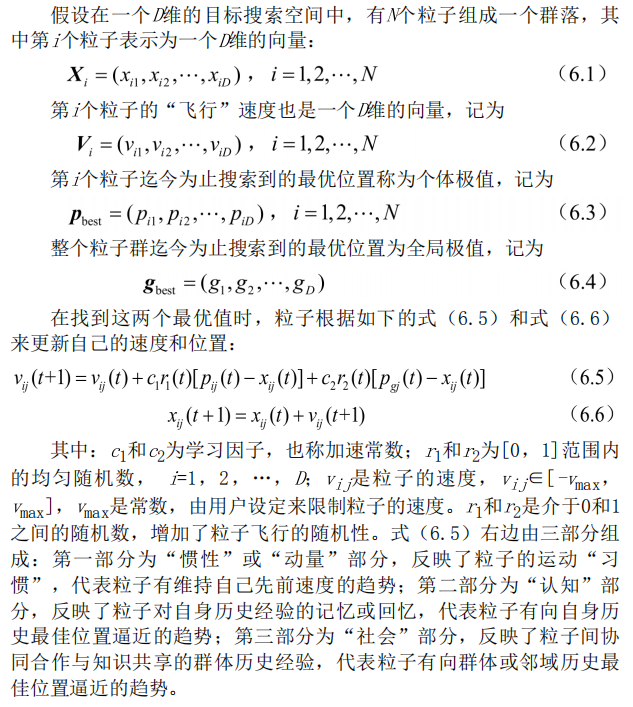3.2标准粒子群算法
引入研究粒子群算法经常用到的两个概念：一是“探索”，指粒子在一定程度上离开原先的搜索轨迹，向新的方向进行搜索，体现了一种向未知区域开拓的能力，类似于全局搜索；二是“开发”，指粒子在一定程度上继续在原先的搜索轨迹上进行更细一步的搜索，主要指对探索过程中所搜索到的区域进行更进一步的搜索。探索是偏离原来的寻优轨迹去寻找一个更好的解，探索能力是一个算法的全局搜索能力。开发是利用一个好的解，继续原来的寻优轨迹去搜索更好的解，它是算法的局部搜索能力。如何确定局部搜索能力和全局搜索能力的比例， 对一个问题的求解过程很重要。1998年， Shi Yuhui等人提出了带有惯性权重的改进粒子群算法，由于该算法能够保证较好的收敛效果，所以被默认为标准粒子群算法。其进化过程为：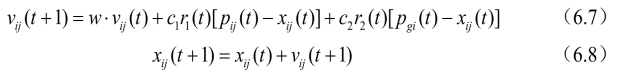在式(6.7)中，第一部分表示粒子先前的速度，用于保证算法的全局收敛性能；第二部分、第三部分则使算法具有局部收敛能力。可以看出，式(6.7)中惯性权重w表示在多大程度上保留原来的速度：W
较大，则全局收敛能力较强，局部收敛能力较弱；w较小，则局部收敛能力较强，全局收敛能力较弱。
当w=1时，式(6.7)与式(6.5)完全一样，表明带惯性权重的粒子群算法是基本粒子群算法的扩展。实验结果表明：w在0.8~1.2之间时，粒子群算法有更快的收敛速度；而当w>1.2时，算法则容易陷入局部极值。
另外，在搜索过程中可以对w进行动态调整：在算法开始时，可给w赋予较大正值，随着搜索的进行，可以线性地使w逐渐减小，这样可以保证在算法开始时，各粒子能够以较大的速度步长在全局范围内探
测到较好的区域；而在搜索后期，较小的w值则保证粒子能够在极值点周围做精细的搜索，从而使算法有较大的概率向全局最优解位置收敛。对w进行调整，可以权衡全局搜索和局部搜索能力。目前，采用较多的动态惯性权重值是Shi提出的线性递减权值策略， 其表达式如下：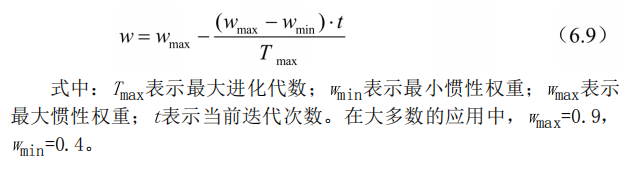3.3压缩因子粒子群算法
Clerc等人提出利用约束因子来控制系统行为的最终收敛 ， 该方法可以有效搜索不同的区域，并且能得到高质量的解。压缩因子法的速度更新公式为：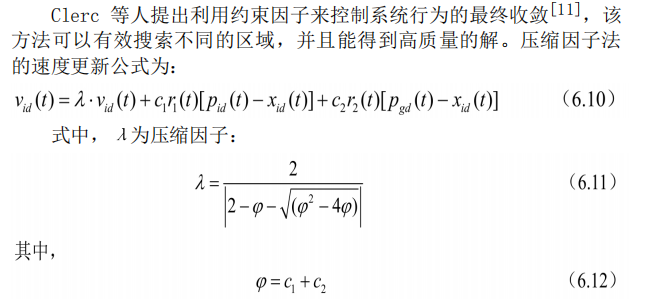实验结果表明：与使用惯性权重的粒子群优化算法相比，使用具
有约束因子的粒子群算法具有更快的收敛速度。
3.4离散粒子群算法
基本的粒子群算法是在连续域中搜索函数极值的有力工具。继基本粒子群算法之后， Kennedy和Eberhart又提出了一种离散二进制版的粒子群算法。在此离散粒子群方法中，将离散问题空间映射到连续粒子运动空间，并适当修改粒子群算法来求解，在计算上仍保留经典粒子群算法速度-位置更新运算规则。粒子在状态空间的取值和变化只限于0和1两个值， 而速度的每一维vi y代表位置每一位xi取值为1的可能性。因此， 在连续粒子群中的vij更新公式依然保持不变， 但是P best和：best只在[0， 1] 内取值。其位置更新等式表示如下：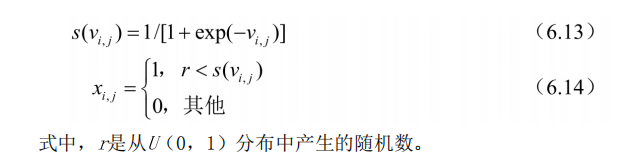4 粒子群算法流程
粒子群算法基于“种群”和“进化”的概念，通过个体间的协作与竞争，实现复杂空间最优解的搜索，其流程如下：
(1)初始化粒子群，包括群体规模N，每个粒子的位置x；和速度Vio
(2) 计算每个粒子的适应度值fit[i] 。
(3) 对每个粒子， 用它的适应度值fit[门和个体极值P best(i)比较。如果fit[i] <P best(i) ， 则用fit[i] 替换掉P best(i) 。
(4) 对每个粒子， 用它的适应度值fit[i] 和全局极值g best比较。如果fit[i] < 8 best， 则用fit[i] 替换g best。
(5)迭代更新粒子的速度v；和位置xj。
(6)进行边界条件处理。
(7)判断算法终止条件是否满足：若是，则结束算法并输出优化结果；否则返回步骤(2)。
粒子群算法的运算流程如图6.1所示。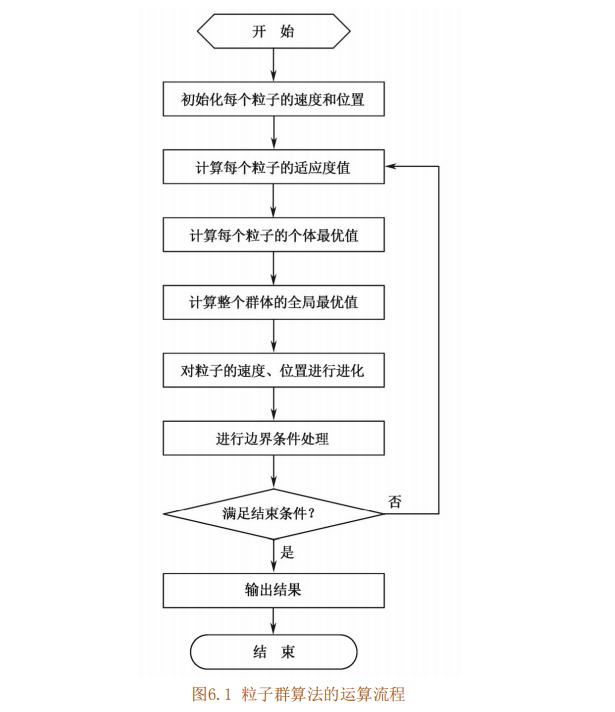5 关键参数说明
在粒子群优化算法中，控制参数的选择能够影响算法的性能和效率；如何选择合适的控制参数使算法性能最佳，是一个复杂的优化问题。在实际的优化问题中，通常根据使用者的经验来选取控制参数。
粒子群算法的控制参数主要包括：粒子种群规模N，惯性权重w，加速系数c和c， 最大速度Via x， 停止准则， 邻域结构的设定， 边界条件处理策略等，
粒子种群规模N
粒子种群大小的选择视具体问题而定，但是一般设置粒子数为20~50。对于大部分的问题10个粒子，已经可以取得很好的结果：不过对于比较难的问题或者特定类型的问题，粒子的数量可以取到100或
200。另外，粒子数目越大，算法搜索的空间范围就越大，也就更容易发现全局最优解；当然，算法运行的时间也越长。
惯性权重w
惯性权重w是标准粒子群算法中非常重要的控制参数，可以用来控制算法的开发和探索能力。惯性权重的大小表示了对粒子当前速度继承的多少。当惯性权重值较大时，全局寻优能力较强，局部寻优能力
较弱：当惯性权重值较小时，全局寻优能力较弱，局部寻优能力较强。惯性权重的选择通常有固定权重和时变权重。固定权重就是选择常数作为惯性权重值，在进化过程中其值保持不变，一般取值为
[0.8，1.2]：时变权重则是设定某一变化区间，在进化过程中按照某种方式逐步减小惯性权重。时变权重的选择包括变化范围和递减率。固定的惯性权重可以使粒子保持相同的探索和开发能力，而时变权重可以使粒子在进化的不同阶段拥有不同的探索和开发能力。
加速常数c1和c2
加速常数c和c 2分别调节向P best和g best方向飞行的最大步长， 它们分别决定粒子个体经验和群体经验对粒子运行轨迹的影响，反映粒子群之间的信息交流。如果cr=c2=0，则粒子将以当前的飞行速度飞到边界。此时，粒子仅能搜索有限的区域，所以难以找到最优解。如果q=0，则为“社会”模型，粒子缺乏认知能力，而只有群体经验，它的收敛速度较快，但容易陷入局部最优；如果oy=0，则为“认知”模
型，没有社会的共享信息，个体之间没有信息的交互，所以找到最优解的概率较小，一个规模为D的群体等价于运行了N个各行其是的粒子。因此一般设置c1=C2，通常可以取c1=cg=1.5。这样，个体经验和群体经验就有了同样重要的影响力，使得最后的最优解更精确。
粒子的最大速度vmax
粒子的速度在空间中的每一维上都有一个最大速度限制值vd max，用来对粒子的速度进行钳制， 使速度控制在范围[-Vimax， +va max] 内，这决定问题空间搜索的力度， 该值一般由用户自己设定。Vmax是一个非常重要的参数，如果该值太大，则粒子们也许会飞过优秀区域：而如果该值太小，则粒子们可能无法对局部最优区域以外的区域进行充分的探测。它们可能会陷入局部最优，而无法移动足够远的距离而跳出局部最优， 达到空间中更佳的位置。研究者指出， 设定Vmax和调整惯性权重的作用是等效的， 所以!max一般用于对种群的初始化进行设定， 即将vmax设定为每维变量的变化范围， 而不再对最大速度进行细致的选择和调节。
停止准则
最大迭代次数、计算精度或最优解的最大停滞步数▲t(或可以接受的满意解)通常认为是停止准则，即算法的终止条件。根据具体的优化问题，停止准则的设定需同时兼顾算法的求解时间、优化质量和
搜索效率等多方面性能。
邻域结构的设定
全局版本的粒子群算法将整个群体作为粒子的邻域，具有收敛速度快的优点，但有时算法会陷入局部最优。局部版本的粒子群算法将位置相近的个体作为粒子的邻域，收敛速度较慢，不易陷入局部最优
值。实际应用中，可先采用全局粒子群算法寻找最优解的方向，即得到大致的结果，然后采用局部粒子群算法在最优点附近进行精细搜索。
边界条件处理
当某一维或若干维的位置或速度超过设定值时，采用边界条件处理策略可将粒子的位置限制在可行搜索空间内，这样能避免种群的膨胀与发散，也能避免粒子大范围地盲目搜索，从而提高了搜索效率。
具体的方法有很多种， 比如通过设置最大位置限制Xmax和最大速度限制Vmax， 当超过最大位置或最大速度时， 在范围内随机产生一个数值代替，或者将其设置为最大值，即边界吸收。

## 二、SVM简介

支持向量机(Support Vector Machine)是Cortes和Vapnik于1995年首先提出的，它在解决小样本、非线性及高维模式识别中表现出许多特有的优势，并能够推广应用到函数拟合等其他机器学习问题中。
1 数学部分
1.1 二维空间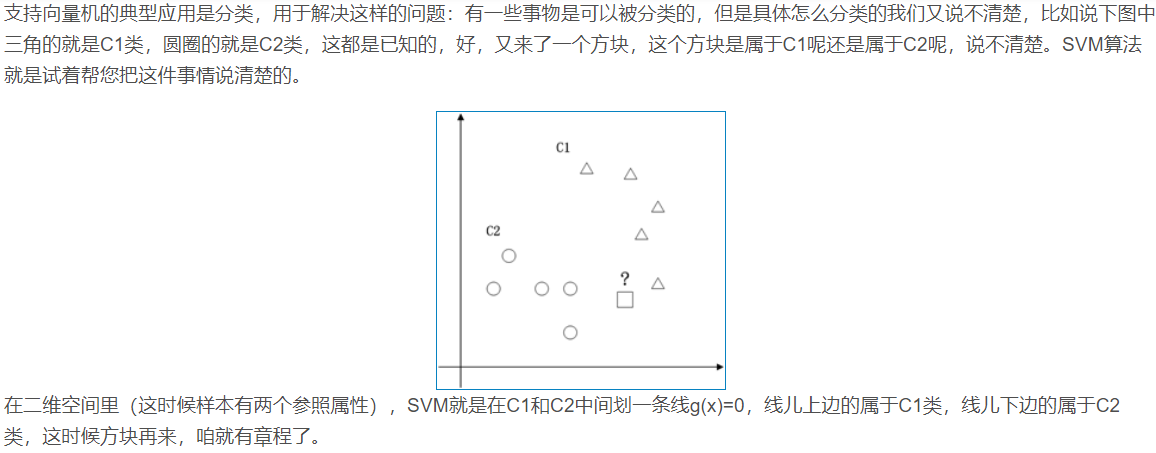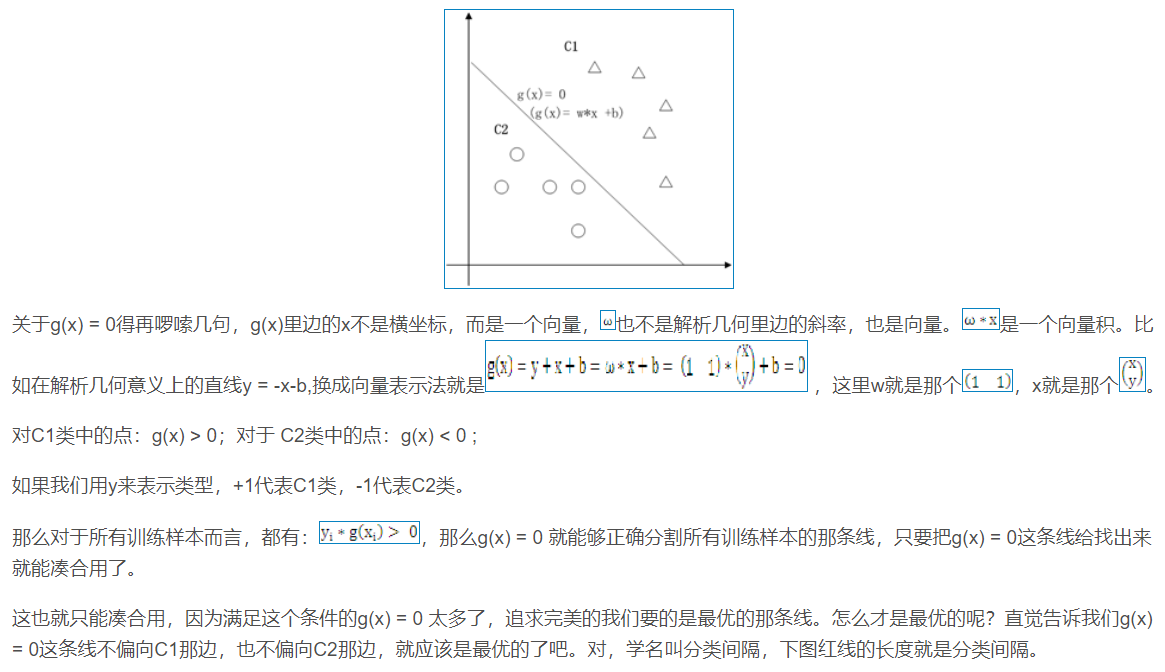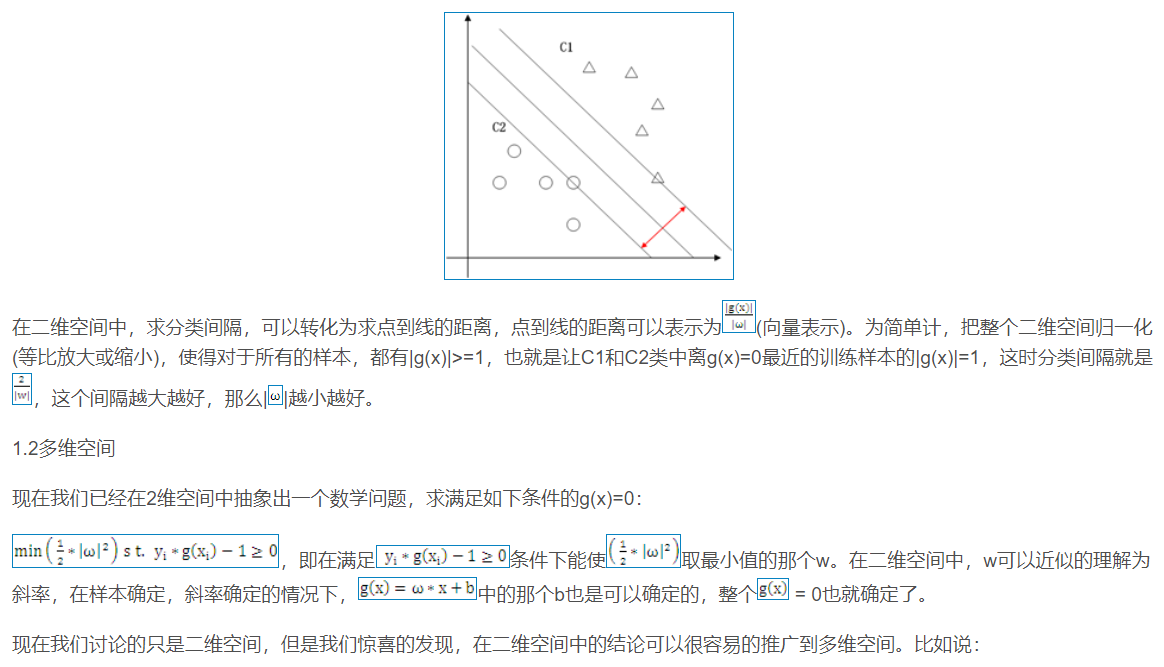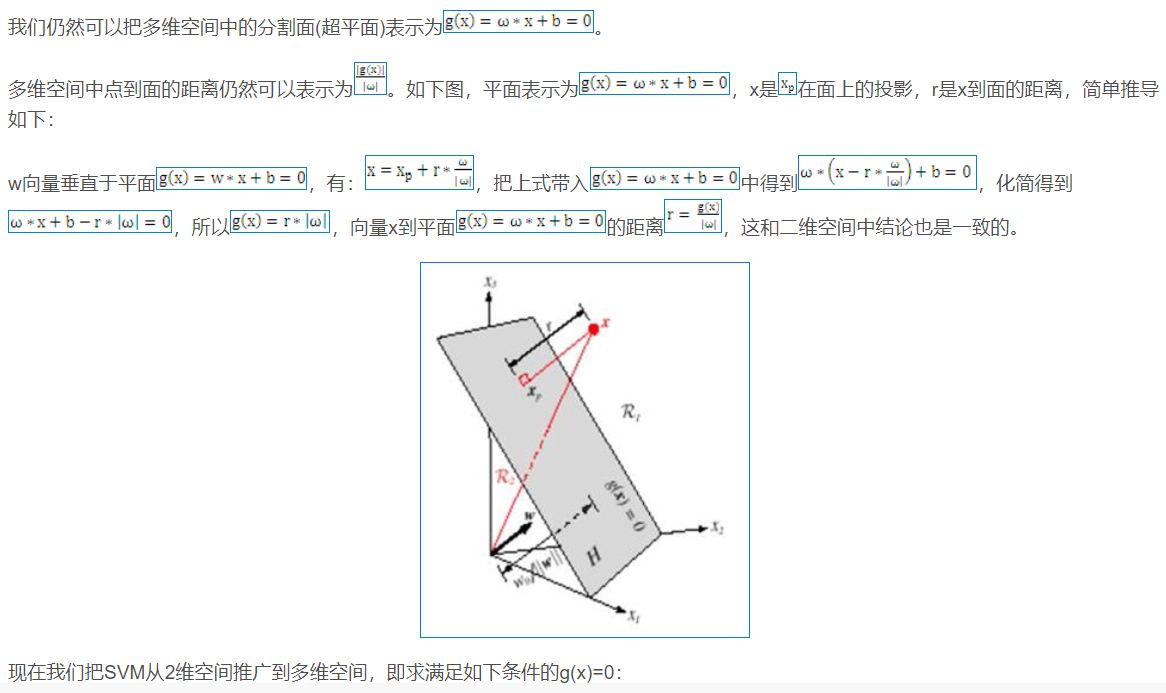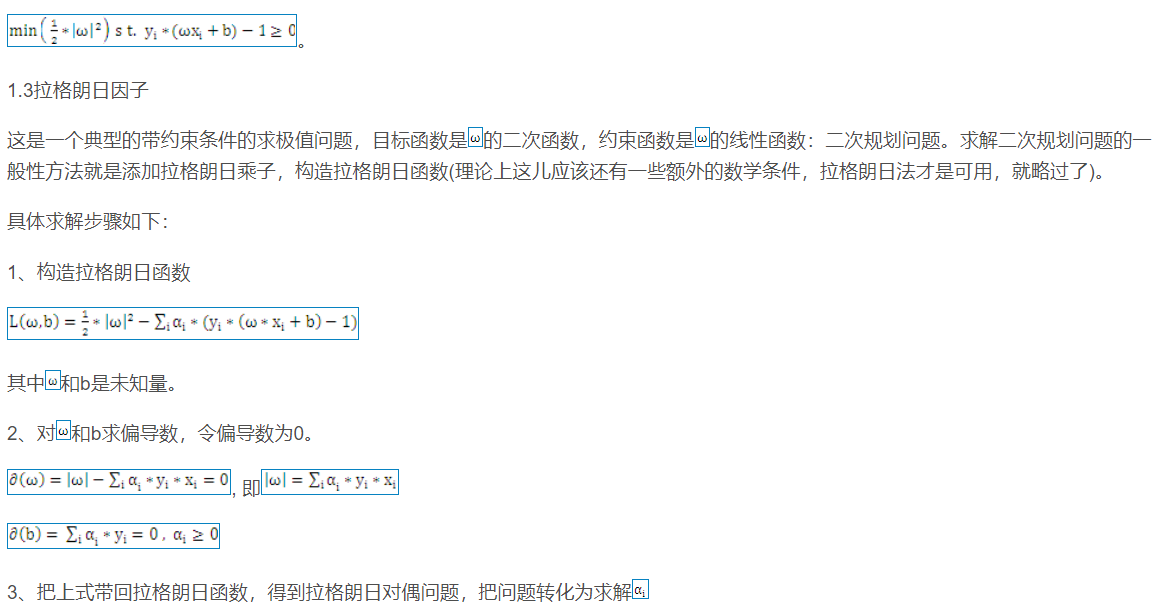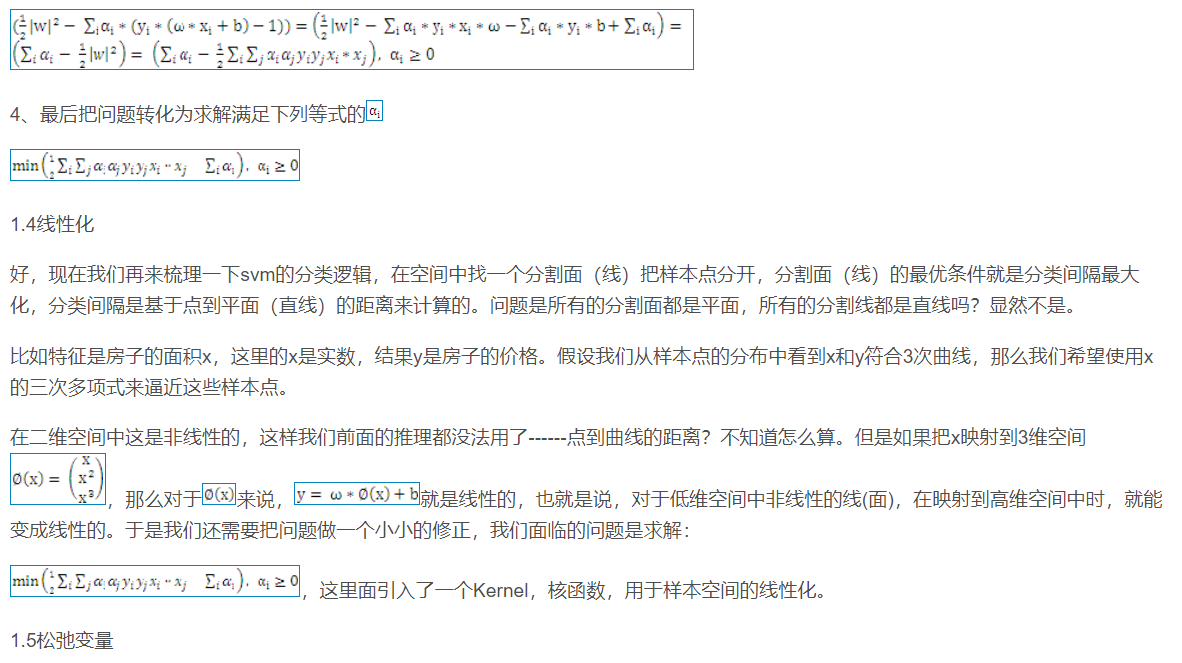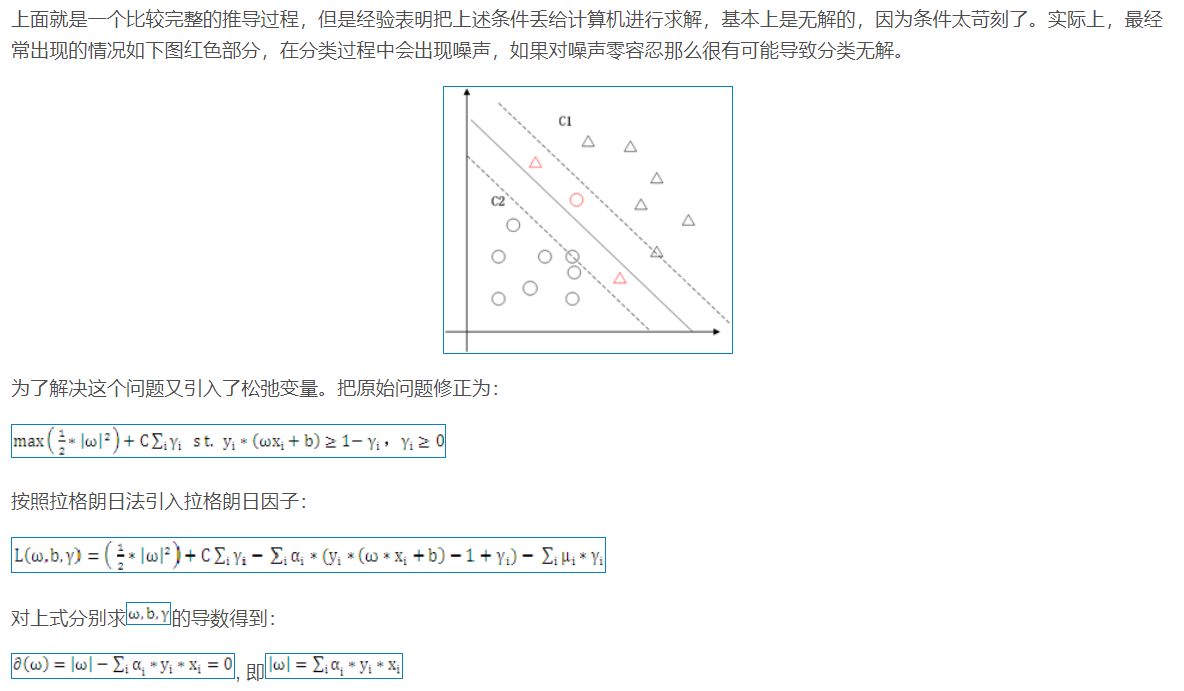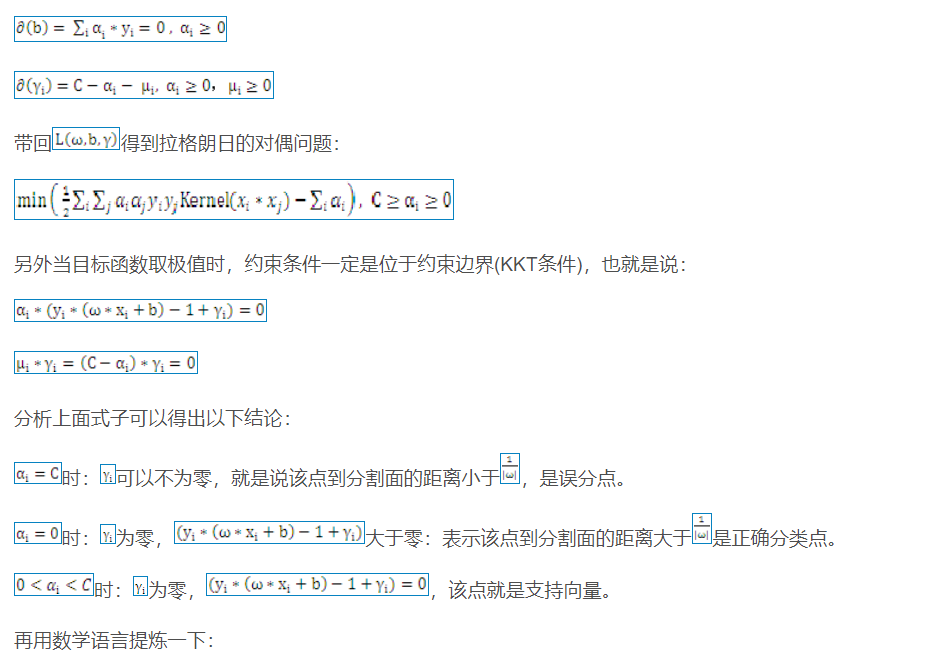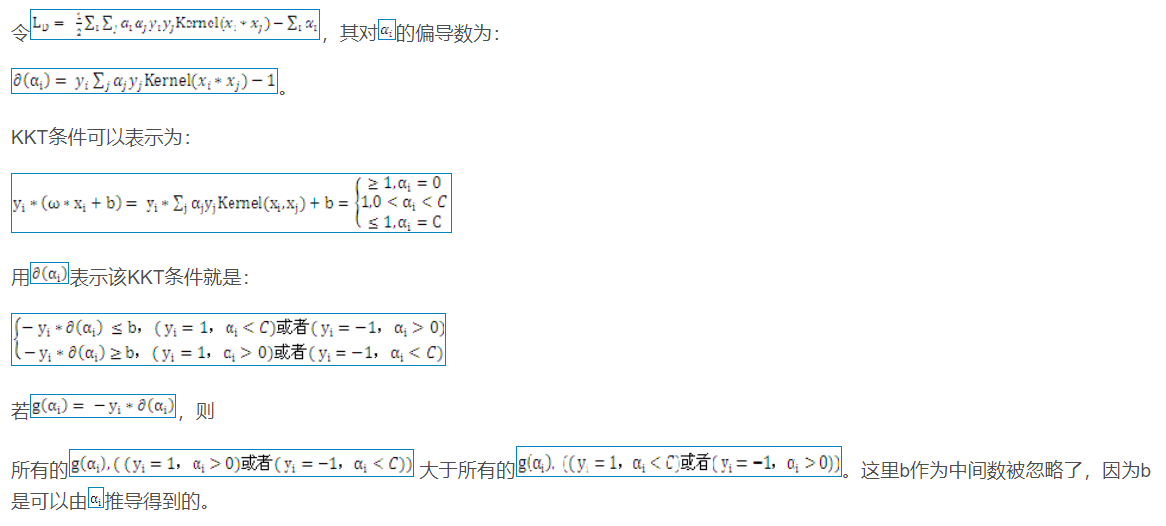2 算法部分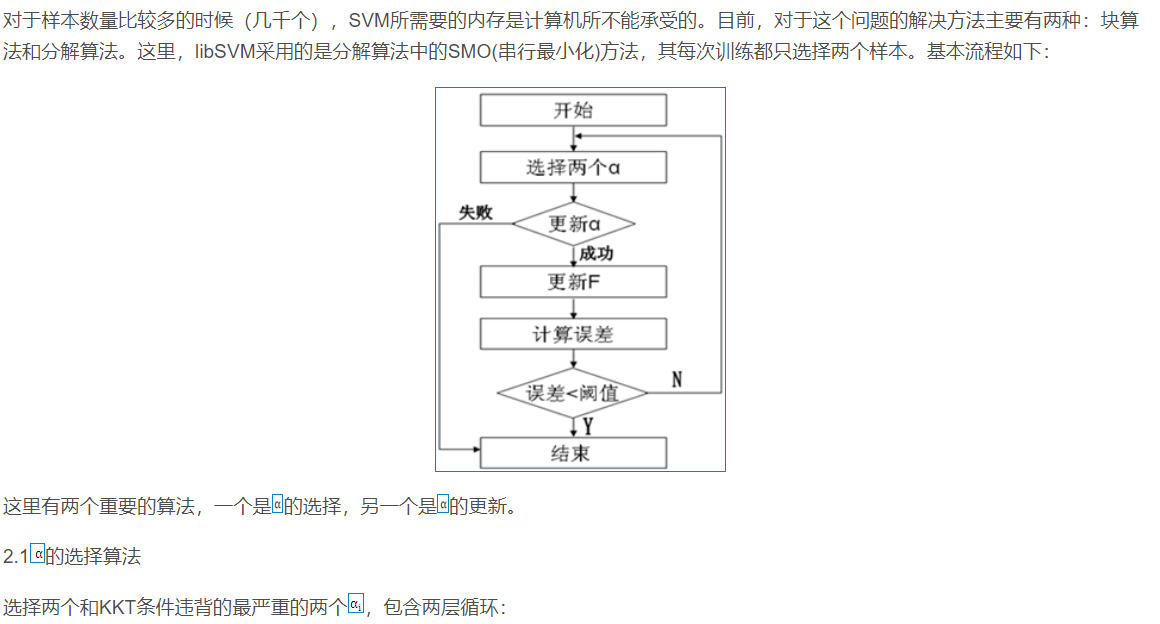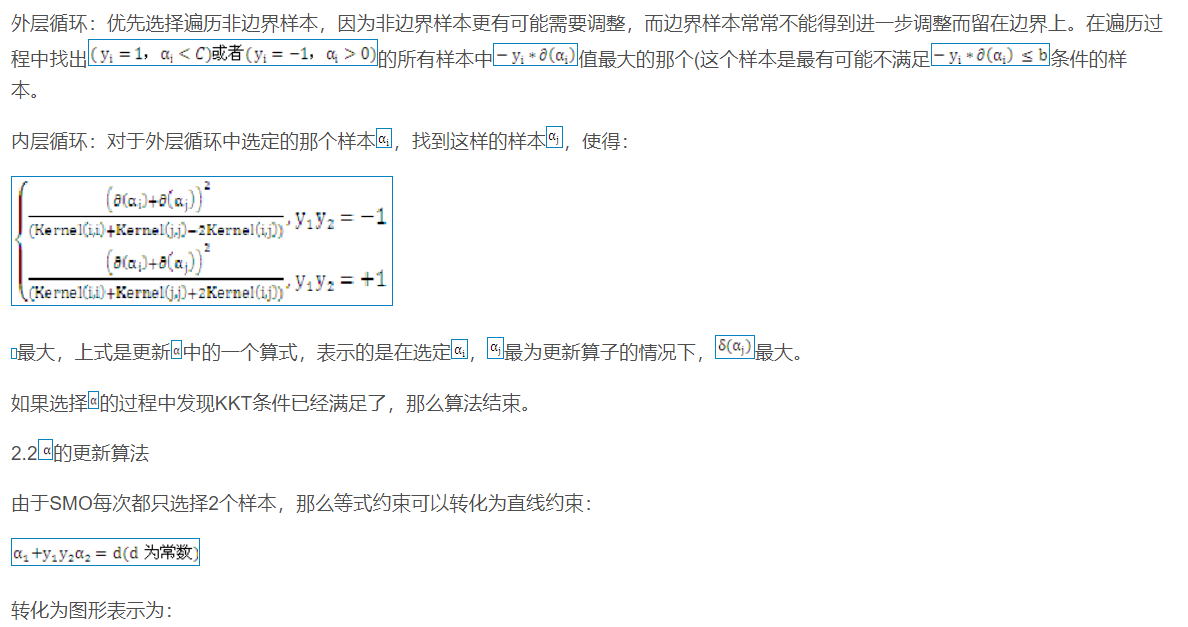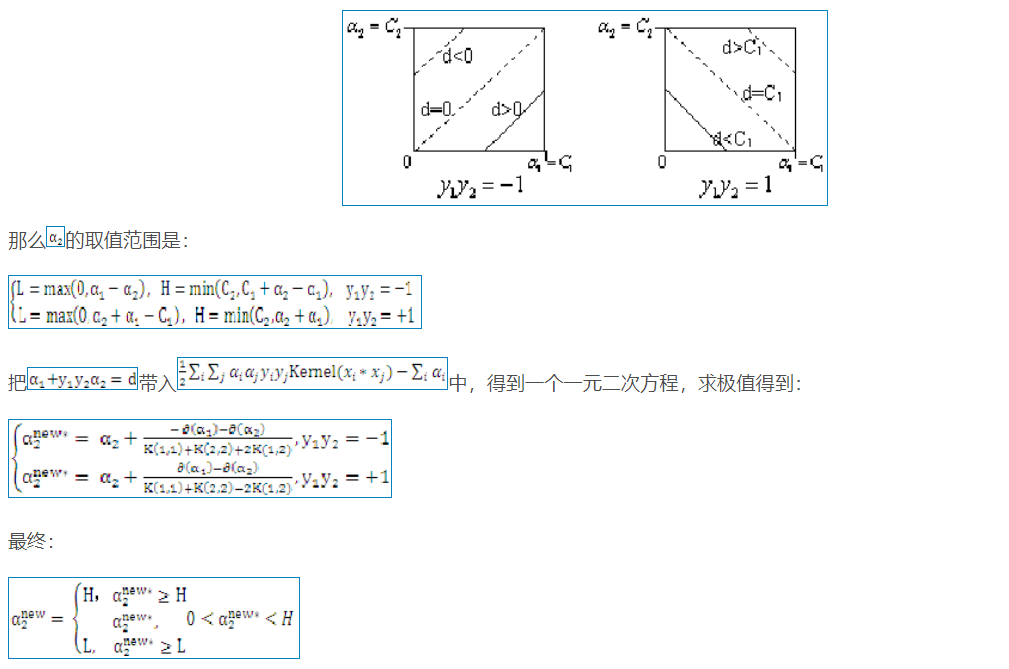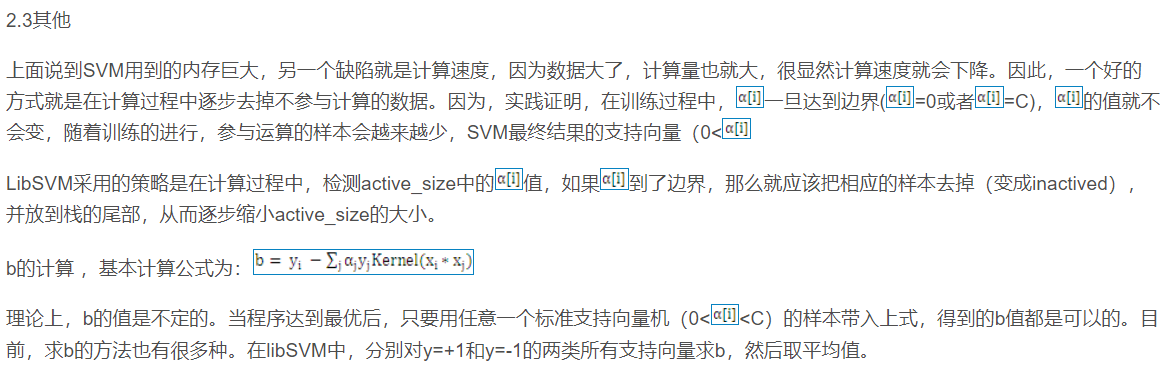## 二、部分源代码

function [bestCVmse,bestc,bestg,fit_gen] = psoSVMcgForRegress(train_label,train,T_test,P_test,pso_option)

%% 参数初始化
% c1:pso参数局部搜索能力
% c2:pso参数全局搜索能力
% maxgen:最大进化数量
% sizepop:种群最大数量
% k:k belongs to [0.1,1.0],速率和x的关系(V = kX)
% wV:(wV best belongs to [0.8,1.2]),速率更新公式中速度前面的弹性系数
% wP:种群更新公式中速度前面的弹性系数

% popcmax:SVM 参数c的变化的最大值.
% popcmin:SVM 参数c的变化的最小值.
% popgmax:SVM 参数g的变化的最大值.
% popgmin:SVM 参数c的变化的最小值.
% popkernel:SVM的核参数

Vcmax = pso_option.k*pso_option.popcmax;
Vcmin = -Vcmax ;
Vgmax = pso_option.k*pso_option.popgmax;
Vgmin = -Vgmax ;
%% 产生初始粒子和速度
for i=1:pso_option.sizepop
% 随机产生种群和速度
i
pop(i,1) = (pso_option.popcmax-pso_option.popcmin)*rand+pso_option.popcmin;
pop(i,2) = (pso_option.popgmax-pso_option.popgmin)*rand+pso_option.popgmin;
V(i,1)=Vcmax*rands(1,1);
V(i,2)=Vgmax*rands(1,1);

% 计算初始适应度
cmd = ['-s 3 -t ',num2str( pso_option.popkernel ),' -c ',num2str( pop(i,1) ),' -g ',num2str( pop(i,2) ),' -p 0.01 -d 1'];
model= svmtrain(train_label, train, cmd);
[l,~]= svmpredict(T_test,P_test,model);
fitness(i)=mse(l-T_test);%以均方差作为适应度函数，均方差越小，精度越高
end

% 找极值和极值点
[global_fitness bestindex]=min(fitness); % 全局极值
local_fitness=fitness;   % 个体极值初始化

global_x=pop(bestindex,:);   % 全局极值点
local_x=pop;    % 个体极值点初始化

% 每一代种群的平均适应度
avgfitness_gen = zeros(1,pso_option.maxgen);

%% 迭代寻优
for i=1:pso_option.maxgen
iter=i
for j=1:pso_option.sizepop

%速度更新
V(j,:) = pso_option.wV*V(j,:) + pso_option.c1*rand*(local_x(j,:) - pop(j,:)) + pso_option.c2*rand*(global_x - pop(j,:));
% 边界判断
if V(j,1) > Vcmax
V(j,1) = Vcmax;
end
if V(j,1) < Vcmin
V(j,1) = Vcmin;
end
if V(j,2) > Vgmax
V(j,2) = Vgmax;
end
if V(j,2) < Vgmin
V(j,2) = Vgmin;
end

%种群更新
pop(j,:)=pop(j,:) + pso_option.wP*V(j,:);
%边界判断
if pop(j,1) > pso_option.popcmax
pop(j,1) = (pso_option.popcmax-pso_option.popcmin)*rand+pso_option.popcmin;;
end
if pop(j,1) < pso_option.popcmin
pop(j,1) = (pso_option.popcmax-pso_option.popcmin)*rand+pso_option.popcmin;;
end
if pop(j,2) > pso_option.popgmax
pop(j,2) = (pso_option.popgmax-pso_option.popgmin)*rand+pso_option.popgmin;;
end
if pop(j,2) < pso_option.popgmin
pop(j,2) = (pso_option.popgmax-pso_option.popgmin)*rand+pso_option.popgmin;;
end

% 自适应粒子变异
if rand>0.8

pop(j,k) = (pso_option.popcmax-pso_option.popcmin)*rand + pso_option.popcmin;
end
if k == 2
pop(j,k) = (pso_option.popgmax-pso_option.popgmin)*rand + pso_option.popgmin;
end
end

%适应度值
cmd = ['-t ',num2str( pso_option.popkernel ),' -c ',num2str( pop(j,1) ),' -g ',num2str( pop(j,2) ),' -s 3 -p 0.01 -d 1'];
model= svmtrain(train_label, train, cmd);
[l,mse1]= svmpredict(T_test,P_test,model);
fitness(j)=mse(l-T_test);
%个体最优更新
if fitness(j) < local_fitness(j)
local_x(j,:) = pop(j,:);
local_fitness(j) = fitness(j);
end

if fitness(j) == local_fitness(j) && pop(j,1) < local_x(j,1)
local_x(j,:) = pop(j,:);
local_fitness(j) = fitness(j);
end

%群体最优更新
if fitness(j) < global_fitness

end

fit_gen(i)=global_fitness;
avgfitness_gen(i) = sum(fitness)/pso_option.sizepop;
end

%% 输出结果
% 最好的参数
bestc = global_x(1);
bestg = global_x(2);
bestCVmse = fit_gen(pso_option.maxgen);%最好的结果

% 支持向量机用于收盘价预测，首先是未优化的，其次是优化后的
%% 清空环境
tic;clc;clear;close all;format compact
%% 加载数据
% 归一化
[a,inputns]=mapminmax(data',0,1);%归一化函数要求输入为行向量
data_trans=data_process(5,a);%% 对时间序列预测建立滚动序列，即用1到m个数据预测第m+1个数据，然后用2到m+1个数据预测第m+2个数据
input=data_trans(:,1:end-1);
output=data_trans(:,end);
%% 数据集 前75%训练 后25%预测
m=round(size(data_trans,1)*0.75);
Pn_train=input(1:m,:);
Tn_train=output(1:m,:);
Pn_test=input(m+1:end,:);
Tn_test=output(m+1:end,:);

%% 1.没有优化的SVM
bestc=0.001;bestg=10;%c和g随机赋值 表示没有优化的SVM
t=0;%t=0为线性核函数,1-多项式。2rbf核函数
cmd = ['-s 3 -t ',num2str(t),' -c ', num2str(bestc),' -g ',num2str(bestg),' -p 0.01 -d 1'];

model = svmtrain(Tn_train,Pn_train,cmd);%训练
[predict,~]= svmpredict(Tn_test,Pn_test,model);%测试
% 反归一化，为后面的结果计算做准备
predict0=mapminmax('reverse',predict',inputns);%测试实际输出反归一化
T_test=mapminmax('reverse',Tn_test',inputns);%测试集期望输出反归一化
T_train=mapminmax('reverse',Tn_train',inputns);%训练集期望输出反归一化



## 三、运行结果## 四、matlab版本及参考文献

1 matlab版本
2014a

2 参考文献
 包子阳,余继周,杨杉.智能优化算法及其MATLAB实例（第2版）[M].电子工业出版社，2016.
张岩,吴水根.MATLAB优化算法源代码[M].清华大学出版社，2017.
周品.MATLAB 神经网络设计与应用[M].清华大学出版社，2013.
陈明.MATLAB神经网络原理与实例精解[M].清华大学出版社，2013.
方清城.MATLAB R2016a神经网络设计与应用28个案例分析[M].清华大学出版社，2018.

展开全文matlab 算法 支持向量机
• matlab支持向量机可以对模型进行预测，具有良好的预测效果
• 支持向量机回归模型，可应用于经济、金融时间序列预测
• svm预测matlab代码支持向量机算法 支持向量机.py： 输入：文档词矩阵 输出：训练模型模型预测 概述：包含用于构建、训练和预测给定数据集的 svm 类。 它还具有创建混淆矩阵的功能 套餐： 需要以下软件包： 用于...
• 智能优化算法、神经网络预测、信号处理、元胞自动机、图像处理、路径规划、无人机等多种领域的Matlab仿真代码matlab
• MATLAB CNN-SVM分类程序，含详细注释及数据，直接根据注释修改自己的文件就可。 以改进VGG网络为例，提取某一网络的某一层特征并用SVM完成分类。 以手写字体识别为例)，识别率可达100...fc1转换为特征向量之后用SVM预测
• 预测模型】基于蝙蝠算法改进SVM实现预测matlab源码.zip简介
• MATLAB实现SVM模型建立，可以模拟预测（包含BP神经网络）
• 针对SVM预测模型参数难以确定的问题,采用SSA算法对SVM中惩罚因子及核函数参数进行优化,构建SSA-SVM股价预测模型。 2 部分代码 clc;clear all;close all;[f p]=uigetfile('*');X=importdata([p f]);...算法 支持向量机 matlab
• 一、麻雀算法优化SVM简介 1 SSA的基本原理 SSA算法是一种模拟麻雀觅食行为和反捕食行为的新型群体智能优化算法，其基本原理如下： 在SSA中，每只麻雀位置对应其中的一个解。麻雀在觅食过程中有3种行为：①作为发现者...matlab 支持向量机 回归
• 预测模型】基于混沌灰狼算法优化支持向量机SVM实现分类matlab源码.zip简介
• 为了提高支持向量机(SVM)模型的拟合精度和泛化能力,以最小化输出量的均方误差为目标,采用基于万有引力定律的优化机制,提出了一种基于引力搜索算法的SVM参数优化方法.通过仿真实验验证,基于引力搜索算法的SVM回归模型...matlab 支持向量机 回归
• svm算法手写matlab代码Matlab机器学习工具集 该代码包含用MATLAB编写的机器学习算法和演示，并用于完成Stanfords机器学习课程。 工作总结： 表示我完成的程序功能。 示例1：假设您是大学部门的管理员，并且您想根据...
•SVM
• 一、混沌灰狼算法简介 1 Tent混沌反向学习策略 为保持种群多样性和使初始化种群个体尽可能均匀分布。在目前文献中,采用较多...基于混沌灰狼优化算法的氧化铝质量指标预测模型[J].广西大学学报(自然科学版). 2016,41(06)matlab 算法 支持向量机
• 预测模型】基于灰狼算法优化支持向量机SVM实现分类matlab源码.zip简介
• 智能优化算法、神经网络预测、信号处理、元胞自动机、图像处理、路径规划、无人机等多种领域的Matlab代码模型及运行结果matlab
• 模型参考这里。 2 代码 %遗传算法主程序 %Name:genmain05.m clear clf popsize=20; %群体大小 chromlength=10; %字符串长度（个体长度） pc=0.6; %交叉概率 pm=0.001; %变异概率 ​ pop=initpop(popsize,...机器学习 python
• 针对SVM预测模型参数难以确定的问题,采用COA算法对SVM中惩罚因子及核函数参数进行优化,构建COA-SVM股价预测模型。 支持向量机是利用已知数据类别的样本为训练样本，寻找同类数据的空间聚集特征，从而对测试样本进行...matlab...

# svm预测模型matlabmatlab 订阅